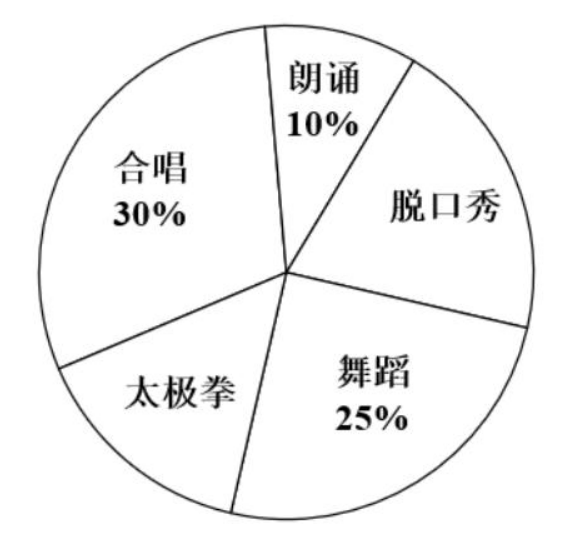$\text{A.}$ 这五个社团的总人数为 100 $\text{B.}$ 脱口秀社团的人数占五个社团总人数的 $20 \%$ $\text{C.}$ 这五个社团总人数占该校学生人数的 $8 \%$ $\text{D.}$ 从这五个社团中任选一人, 其来脱口秀社团或舞蹈社团的概率为 $50 \%$